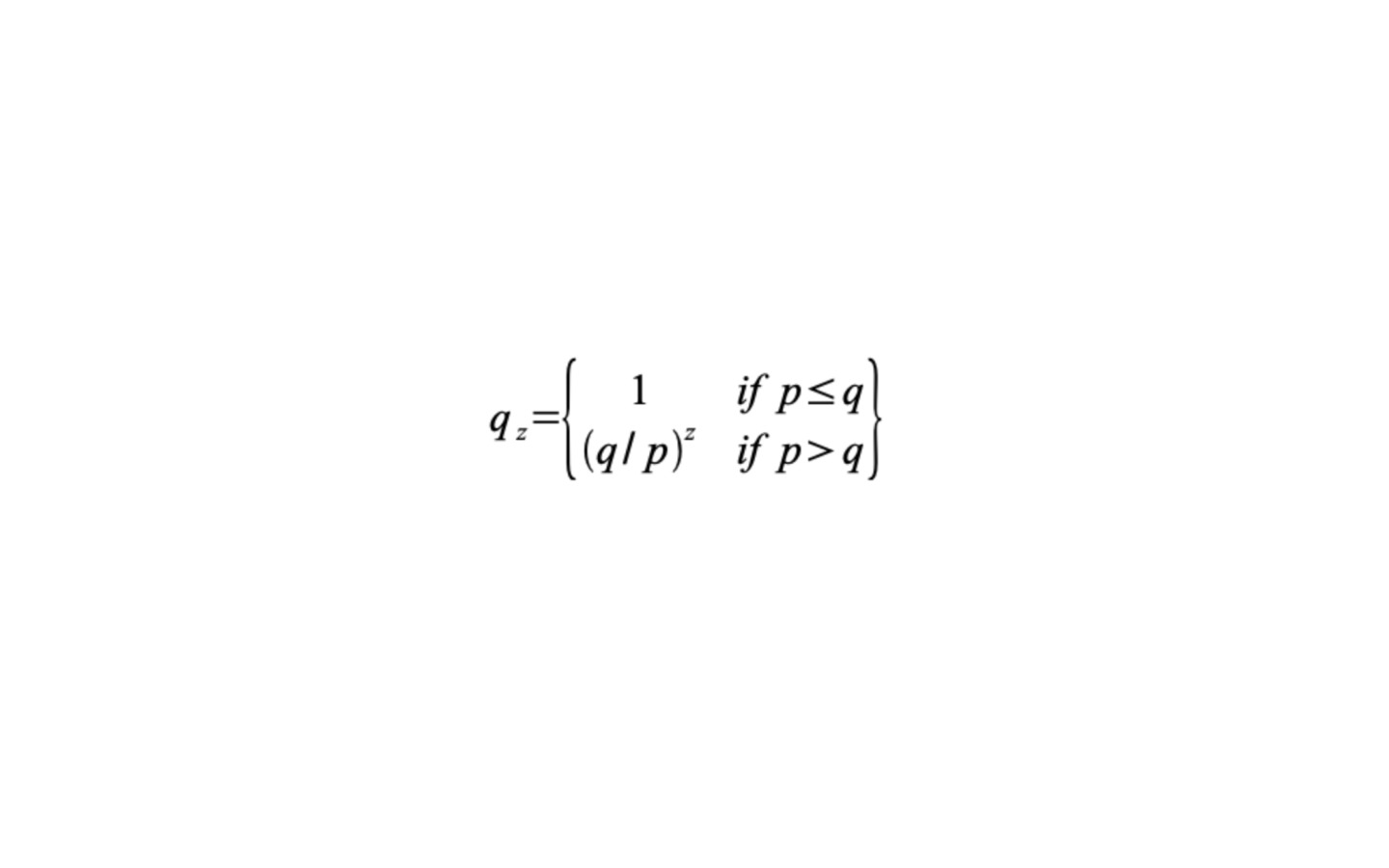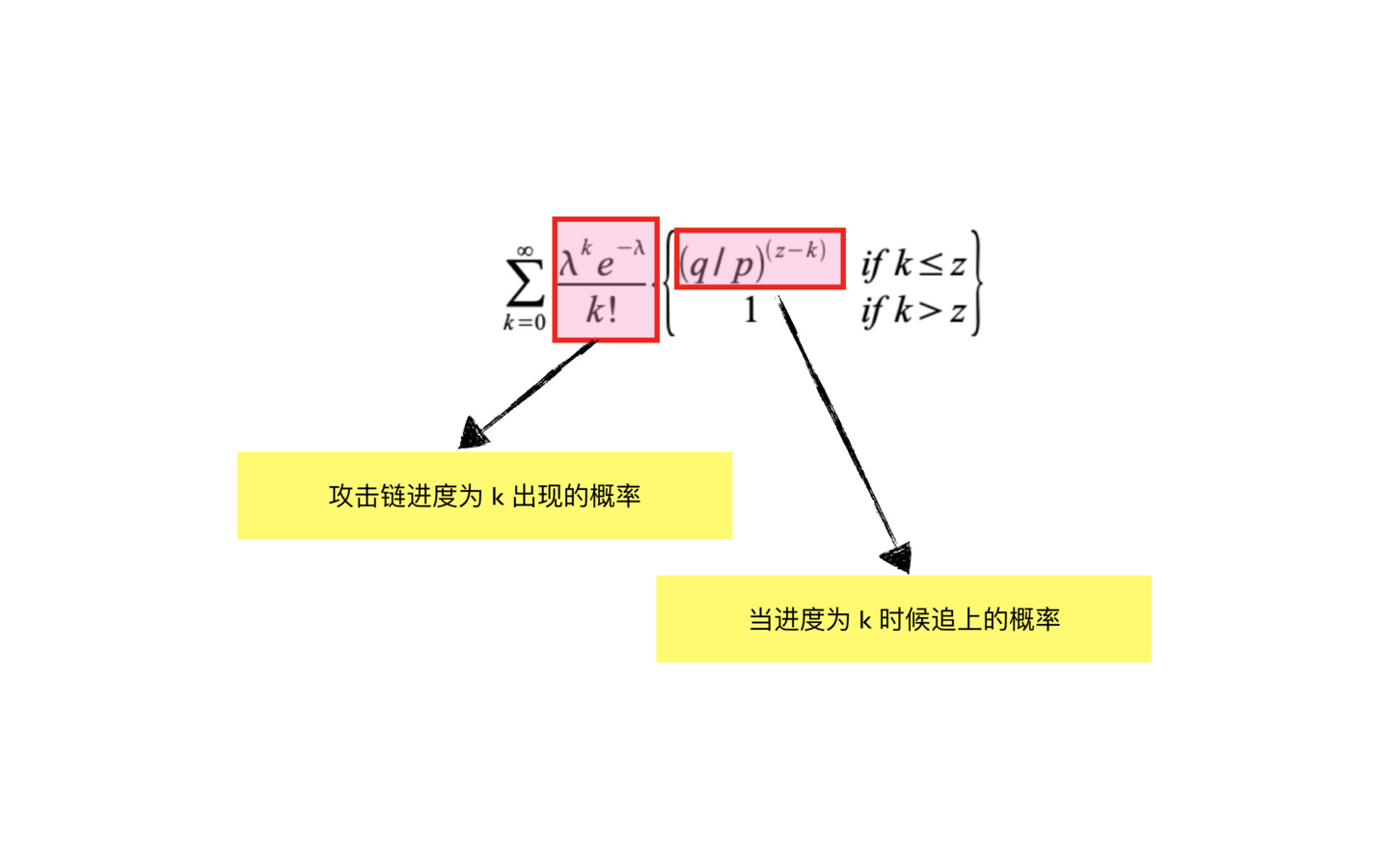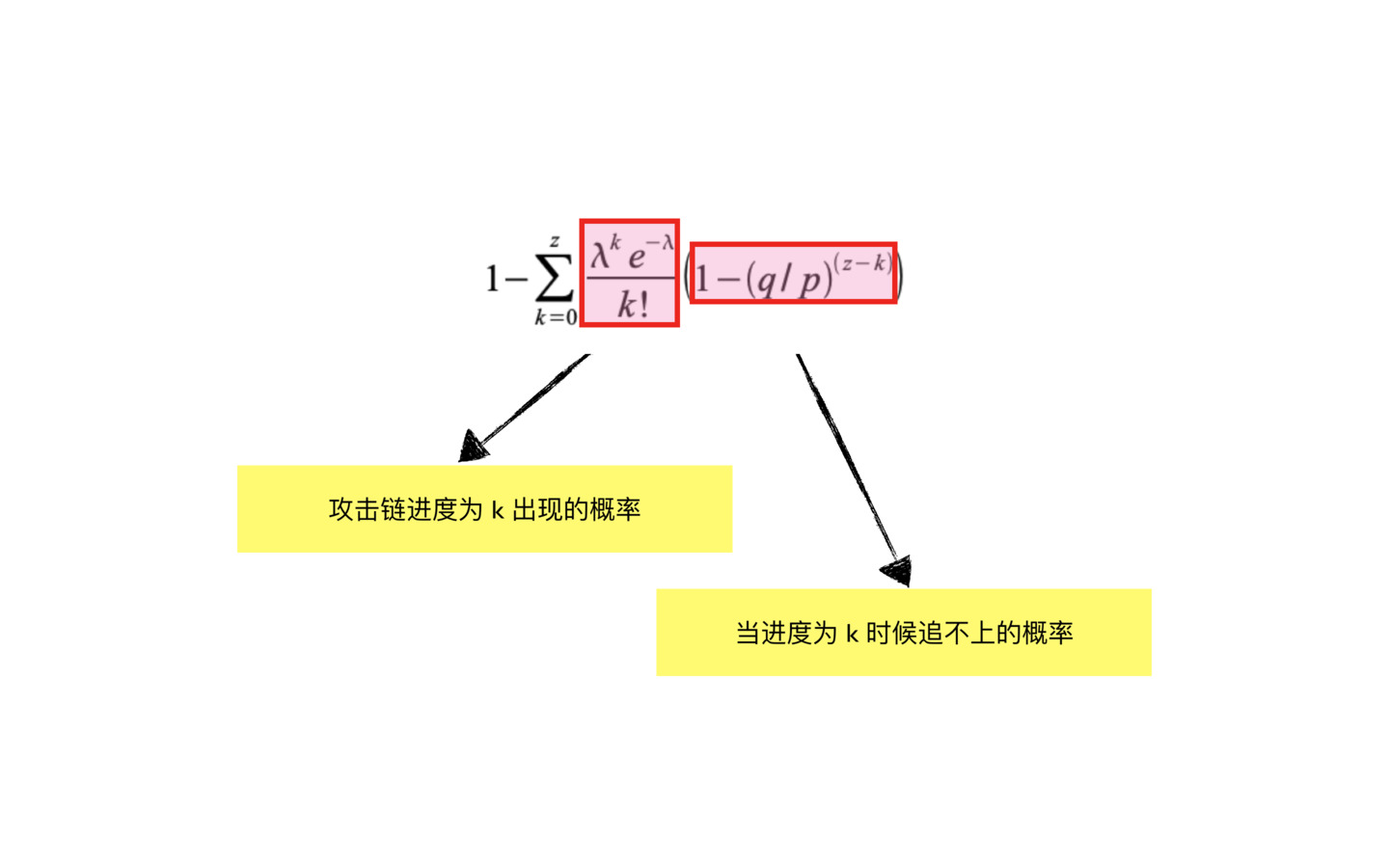# BTC White Paper解讀

## 比特幣如何做到

In this paper, we propose a solution to the double-spending problem using a peer-to-peer distributed timestamp server to generate computational proof of the chronological order of transactions

## 比特幣的交易機制(避免雙花機制)

define an electronic coin as a chain of digital signatures### Timestamp Server### Proof-of-Work### Network

1. 新交易廣播到全部的節點(nodes)
2. 每個節點收集交易打包成block
3. 每個節點計算nonce去找到proof-of-work for this block
4. 一旦找到了proof-of-work, 就廣播給其他節點
5. 節點驗證該區塊的交易是否都有效
6. 節點會幫忙散播此區塊, 因為他們想要創建下一個區塊也是根據此區塊創建的, 所以必須幫助此鏈獲得最長鏈

### Reclaiming Disk Space### Full node

Full node即儲存所有交易訊息的節點, 比特幣建議的full node的規格如下

• 200GB的儲存空間, 讀寫速度要在100MB以上
• 2GB以上的記憶體
• 上傳速度400Kbps的網路

### SPV(Simplified Payment Verification) node## Combining and Splitting Value

### Privacy## Calculations

### Poisson Distribution

(1) 在一個短時間區間 $\Delta t$ 內，發生一次事件的機率與 $\Delta t$ 成正比： $\lambda \Delta t$。(代表$\Delta t \lambda$為均值得概念)
(2) 在短時間內發生兩次以上的機率可以忽略。(可以想成機率函數介於0~1)
(3) 在不重疊的時間段落裡，事件各自發生的次數是獨立的。(每一次$\Delta t$是獨立事件)

$P(k, T) ~ C^{N}_{k}(\lambda \Delta t)^{k}(1-\lambda\Delta t)^{N-k}$

$=\frac{N(N-1)...(N-k+1)}{k!}\lambda^k\frac{T^k}{N^k}\frac{(1-\frac{\lambda T}{N})^N}{(1-\frac{\lambda T}{N})^k}$

$=\frac{(\lambda T)^k}{k!}\frac{N(N-1)...(N-k+1)}{N N N...N}\frac{(1-\frac{\lambda T}{N})^N}{(1-\frac{\lambda T}{N})^k}$

$P(X=k) = \frac{e^{-\lambda}\lambda^{k}}{k!}$

http://episte.math.ntu.edu.tw/articles/sm/sm_16_07_1/index.html

## Attacker追上交易的機率

1. Attacker產生k個block的機率
2. Attacker產生k個block追上honest z個block的機率

p + q = 1

### Attacker 產生k個block追上honest z個block的機率### Attacker 產生k個block的機率

$\lambda = z\frac{q}{p}$

$\frac{\lambda^ke^{-\lambda}}{k!}$

### 結合計算#include <math.h>
double AttackerSuccessProbability(double q, int z)
{
double p = 1.0 - q;
double lambda = z * (q / p);
double sum = 1.0;
int i, k;
for (k = 0; k <= z; k++)
{
double poisson = exp(-lambda);
for (i = 1; i <= k; i++)
poisson *= lambda / i;
sum -= poisson * (1 - pow(q / p, z - k));
}
return sum;
}


Solving for P less than 0.1%...

P < 0.001
q=0.10 z=5
q=0.15 z=8
q=0.20 z=11
q=0.25 z=15
q=0.30 z=24
q=0.35 z=41
q=0.40 z=89
q=0.45 z=340

BTC (BTC) : 1LFRBqvWR9GGizBzoFddkb5xRpAzKRVoDC
BTC (BSC) : 0xe1cda3eb778d1751af17feac796a4bbe4dbca815
BTC (ERC20) : 0xe1cda3eb778d1751af17feac796a4bbe4dbca815
USDT (TRC20) : TT7wgKuYoHwfRy3vCr38Qy3gnS3JJ1aKvn